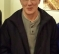## How to use compression to change bit depth of a video signal?Started by 3 years ago5 replieslatest reply 3 years ago203 views
I need to use compression to change bit depth of a video signal.

Our Focal Plane Array(FPA) is Vanadium Oxide(VOx) Resistive Microbolometers Uncooled Thermal Infrared Detector.  The output of this image sensor is 14-bit, Wide Dynamic Range(WDR), raw, monochrome, 640 x 480 resolution.  The pixels of this video signal will be processed by Image Signal Processor(ISP).  ISP input is 12-bits.  Therefore, I need to compress FPA output to 12 bits and then input into ISP.

How to perform global tone mapping using one of two methods, PWL or LUT (curve defined by lut) based mapping.  I need to tone map 14 bit data to 12 bit.  Please provide sufficient information so I can achieve this.  It's suppose to be similar to gamma compression.  I can do gamma correction in matlab.  Can this tone mapping also be done in matlab?  If yes, please provide some examples.
[ - ]One think you didn't state is what sort of input your ISP expects.  If it is expecting raw monochrome video, then the simplest approach would be to just drop the two LSBs of the raw data.

If your ISP can handle preprocessed data, do you have a description of what tone mapping you want to do?  If so, yes, you can do it in Matlab.  You'll have to capture the raw data and feed it into your Matlab code and then output the processed data to your ISP.  It won't be real-time.

[ - ]ISP will treat the input data as 12-bit unsigned. ISP can handle preprocessed data.

Description is global tone mapping can be done using one of two methods:        1.  Piecewise Linear(PWL)                                                       2.  Look-up Table(LUT) based mapping

How to do global tone mapping from 14-bit to 12-bit?  What is  my end goal?  I think my end goal is that 12-bit data should be HDR, similar to 14-bit HDR.  Tone mapped data should be similar to 14-bit data?

What tone map function should I use?  There are basic global tone mapping functions such as logarithm, power function, sigmoid.  Will one of these work or I need a more sophisticated image dependent or image sensor dependent function?

>> then the simplest approach would be to just drop the two LSBs of the raw data.                                                                           Sensor manufacturer says dropping two LSBs will increase Noise Equivalent Temperature Difference(NETD) by a factor of 4.

The raw data that I need to feed into Matlab code should be in what format? Usually we feed input images into Matlab and output is modified image?

[ - ]The output of your micro-bolometer system is 14-bit raw data.  Your ISP needs 12-bit data, but you can use non-linear data.  You spoke of using Matlab to do gamma correction and asked about tone mapping.  Was your idea to use Matlab to do the mapping on a computer or just model the mapping, or to convert the matlab to a program in some other language that will run on a computer (or FPGA)?

Secondly, do you have any information about the input levels?  Are you using the 14-bit dynamic range?  If, as an extreme example, the inputs were almost always in the range of 1000, you could drop the two MSBs and just have any output above 4095 get mapped to 4095.

Given that you need the whole range, jbrower's suggestion of 1 16K lookup table with values scaled to gamma compression values is probably your best choice.

[ - ]Yes, output of micro-bolometer system is 14-bit data.  Global Tone mapping is already implemented in software but this Global Tone mapping operates on a LUT.
Global Tone mapping software input is 14-bit.  It outputs 12-bit.  jbrower solution of 0.857 gamma may be the function I need.
Before deciding on a LUT, I need to understand Global Tone mapping software implementation.

How to determine what LUT or function I need?  Does it depend on image sensor properties and existing global tone mapping implementation?

I want to use matlab to decide which LUT or function I need?  If image sensor manufacturer can provide sensor output images, I can apply different LUTs to it and view the resulting image.

Yes, 14-bit High Dynamic range.

[ - ]